# Martin Orr's Blog

## Weil pairings: the skew-symmetric pairing

Posted by Martin Orr on Tuesday, 06 September 2011 at 13:52

Last time, we defined a pairingBy composing this with a polarisation, we get a pairing ofwith itself. This pairing is symplectic; the proof of this will occupy most of the post.

We will also see that the action of the Galois group on this pairing is given by the (inverse of the) cyclotomic character, as I promised a long time ago (in the comments). This tells us that the image of the-adic Galois representation of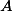is contained in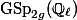. This is the end of my series on Mumford-Tate groups and-adic representations attached to abelian varieties.

### Definition of the pairing

Letbe a polarisation. We definebywhereis the Weil pairing defined last time.

Taking the inverse limit over powers of, we get the-adic pairing### The theta group

We would like to show that the pairingsare symplectic. The elegant way to do this is by introducing the theta group of a line bundle.

Letbe a line bundle on. We define the theta group ofto beThis is a group under the operation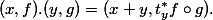The inverse is given by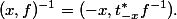(The formula foris functorial in, so really this is not just a group but the functor of points of a group scheme.)

Projection onto the first factor gives a surjection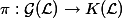where. We are mainly interested in non-degenerate line bundles i.e. those for which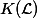is finite; we have already proved that ample line bundles are non-degenerate.

The kernel ofis the group of automorphisms of, which is the scalars. So we have a short exact sequence of group schemes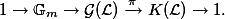This short exact sequence has the following two properties:

1.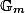is contained in the centre of.
2.is commutative.

These two properties imply that the commutatorof any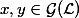lies in, and thatdepends only onand. So the commutator induces a function, and a calculation shows that this is a bihomomorphism.

An explicit calculation of the commutator gives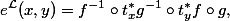whereandare in.

The commutator pairing is obviously symplectic. So in order to show thatis symplectic, it will suffice to relate it to.

Theorem. Let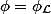be a polarisation. If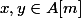andsuch that, thenProof. We must first check that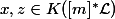so thatis defined. Since, we haveSo. Likewise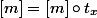so that.

Choose an isomorphism. Thanks to the canonical isomorphismswe can also interpretas an isomorphism. Hence, by the definition of the Weil pairing, we haveSince the line bundlesandare not just isomorphic but equal, we have.

Substitutingand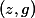in the commutator formula gives### The Galois action

All the pairings we have defined are compatible with the Galois action in that if, then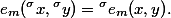Now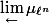is a free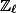-module of rank 1, traditionally written as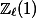. The Galois action on this module is given by a character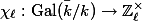called the-adic cyclotomic character. ConcretelyMeanwhile the natural action ofon the spaceof symplectic forms onis given by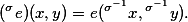So we see thatThe representation ofonpreserves the non-degenerate symplectic pairingup to a scalar, so the image of this representation is contained in. We saw long ago that the Mumford-Tate group is contained in, so this was expected.

1. Tate classes From Martin's Blog

In my last post I talked about Hodge classes on abelian varieties. Today I will talk about the analogue in l-adic cohomology, called Tate classes. These are defined to be those classes on which the action of the Galois group is given by multiplying ...